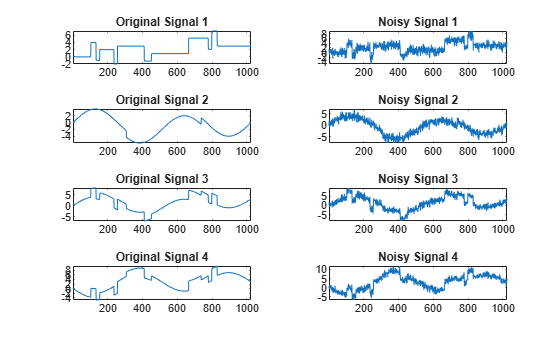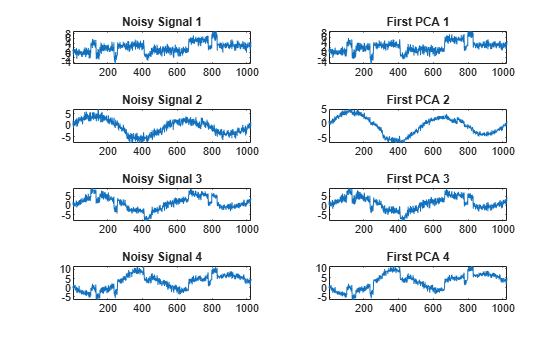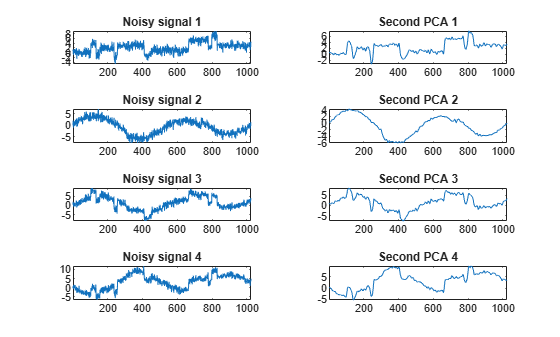# wmspca

Multiscale Principal Component Analysis

## Syntax

```[X_SIM,QUAL,NPC,DEC_SIM,PCA_Params] = wmspca(X,LEVEL,WNAME,NPC) [...] = wmspca(X,LEVEL,WNAME,'mode',EXTMODE,NPC) [...] = wmspca(DEC,NPC) [...] = wmspca(X,LEVEL,WNAME,'mode',EXTMODE,NPC) ```

## Description

`[X_SIM,QUAL,NPC,DEC_SIM,PCA_Params] = wmspca(X,LEVEL,WNAME,NPC)` or `[...] = wmspca(X,LEVEL,WNAME,'mode',EXTMODE,NPC)` returns a simplified version `X_SIM` of the input matrix `X` obtained from the wavelet-based multiscale principal component analysis (PCA).

The input matrix `X` contains `P` signals of length `N` stored column-wise (`N` > `P`).

### Wavelet Decomposition Parameters

The wavelet decomposition is performed using the decomposition level `LEVEL` and the wavelet `WNAME`.

`EXTMODE` is the extended mode for the DWT (See `dwtmode`).

If a decomposition `DEC` obtained using `mdwtdec` is available, you can use

`[...] = wmspca(DEC,NPC)` instead of

`[...] = wmspca(X,LEVEL,WNAME,'mode',EXTMODE,NPC)`.

### Principal Components Parameter: NPC

If `NPC` is a vector, then it must be of length `LEVEL+2`. It contains the number of retained principal components for each PCA performed:

• `NPC(d)` is the number of retained noncentered principal components for details at level `d`, for 1 <= `d` <= `LEVEL`.

• `NPC(LEVEL+1)` is the number of retained non-centered principal components for approximations at level LEVEL.

• `NPC(LEVEL+2)` is the number of retained principal components for final PCA after wavelet reconstruction.

`NPC` must be such that 0 <= `NPC(d)` <= `P` for 1 <= `d` <= `LEVEL`+2.

If `NPC = 'kais'` (respectively, `'heur'`), then the number of retained principal components is selected automatically using Kaiser's rule (or the heuristic rule).

• Kaiser's rule keeps the components associated with eigenvalues greater the mean of all eigenvalues.

• The heuristic rule keeps the components associated with eigenvalues greater than 0.05 times the sum of all eigenvalues.

If `NPC = 'nodet'`, then the details are “killed” and all the approximations are retained.

### Output Parameters

`X_SIM` is a simplified version of the matrix `X`.

`QUAL` is a vector of length `P` containing the quality of column reconstructions given by the relative mean square errors in percent.

`NPC` is the vector of selected numbers of retained principal components.

`DEC_SIM` is the wavelet decomposition of `X_SIM`

`PCA_Params` is a structure array of length `LEVEL+2` such that:

• `PCA_Params(d).pc` is a `P`-by-`P` matrix of principal components.

The columns are stored in descending order of the variances.

• `PCA_Params(d).variances` is the principal component variances vector.

• `PCA_Params(d).npc = NPC`

## Examples

### Wavelet Principal Component Analysis of Noisy Multivariate Signal

Use wavelet multiscale principal component analysis to denoise a multivariate signal.

Load the dataset consisting of four signals of length 1024. Plot the original signals and the signals with additive noise.

```load ex4mwden; kp = 0; for i = 1:4 subplot(4,2,kp+1) plot(x_orig(:,i)) axis tight title(['Original signal ',num2str(i)]) subplot(4,2,kp+2) plot(x(:,i)) axis tight title(['Noisy signal ',num2str(i)]) kp = kp + 2; end```Perform the first multiscale wavelet PCA using the Daubechies least-asymmetric wavelet with four vanishing moments, `sym4`. Obtain the multiresolution decomposition down to level 5. Use the heuristic rule to decide how many principal components to retain.

```level = 5; wname = 'sym4'; npc = 'heur'; [x_sim, qual, npc] = wmspca(x,level,wname,npc);```

Plot the result and examine the quality of the approximation.

`qual`
```qual = 1×4 97.4372 94.5520 97.7362 99.5219 ```
```kp = 0; for i = 1:4 subplot(4,2,kp+1) plot(x(:,i)) axis tight title(['Noisy signal ',num2str(i)]) subplot(4,2,kp+2) plot(x_sim(:,i)) axis tight title(['First PCA ',num2str(i)]) kp = kp+2; end```The quality results are all close to 100%. The `npc` vector gives the number of principal components retained at each level.

Suppress the noise by removing the principal components at levels 1�3. Perform the multiscale PCA again.

```npc(1:3) = zeros(1,3); [x_sim, qual, npc] = wmspca(x,level,wname,npc);```

Plot the result.

```kp = 0; for i = 1:4 subplot(4,2,kp+1) plot(x(:,i)) axis tight title(['Noisy signal ',num2str(i)]) subplot(4,2,kp+2) plot(x_sim(:,i)) axis tight title(['Second PCA ',num2str(i)]) kp = kp+2; end```## Algorithms

The multiscale principal components generalizes the usual PCA of a multivariate signal seen as a matrix by performing simultaneously a PCA on the matrices of details of different levels. In addition, a PCA is performed also on the coarser approximation coefficients matrix in the wavelet domain as well as on the final reconstructed matrix. By selecting conveniently the numbers of retained principal components, interesting simplified signals can be reconstructed.

## References

Aminghafari, M.; Cheze, N.; Poggi, J-M. (2006), “Multivariate de-noising using wavelets and principal component analysis,” Computational Statistics & Data Analysis, 50, pp. 2381–2398.

Bakshi, B. (1998), “Multiscale PCA with application to MSPC monitoring,” AIChE J., 44, pp. 1596–1610.